# JEE Main 2019 Physics Question Paper With Solutions - January Shift

JEE Main 2019 Physics question paper solutions are provided here to help JEE candidates find accurate and detailed solutions for all the problems covered in the January session of the entrance exam. The solutions have been prepared by our subject experts and will help candidates to attain the right approach in solving both conceptual or numerical problems.

The solutions will further help JEE aspirants to prepare effectively for the forthcoming competitive exam. The solutions have been developed with one main aim which is to help students clearly understand and properly evaluate several concepts covered in Physics syllabus. If candidates have any doubts they can use the solution to clear them as well. The solution can be viewed directly on our page or it can be downloaded in a PDF format.

JEE Main 2019 Physics Solutions 9th Jan:-

## JEE Main Jan 2019 Physics Question Paper And Solutions

1. A block of mass 10 kg is kept on a rough incline as shown in the figure. The coefficient of friction between the block and the surface is 0.6. Two forces of magnitudes 3N and P Newton are acting off the block as shown in the figure. If friction on the block is acting upwards then the minimum value of P for which the block remains at rest is: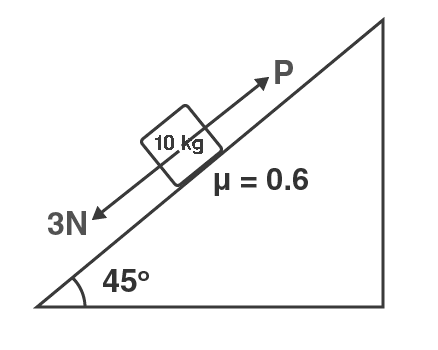(1) 64 N

(2) 32 N

(3) 12 N

(4) 3 N

Solution:

Upward force = Downward force

mg sin θ +3 = p + l

mg sin θ + 3 = P + µ(N)

Substituting,

$\frac{10 \times 10}{\sqrt{2}} + 3 = P + \frac{0.6 \times 10 \times 10}{\sqrt{2}}$

Simplifying P = 32N

2. For path ABC, the heat given to the system is 60 J and work done by the system is 30 J. For path ADC, the work done by the system is 10J.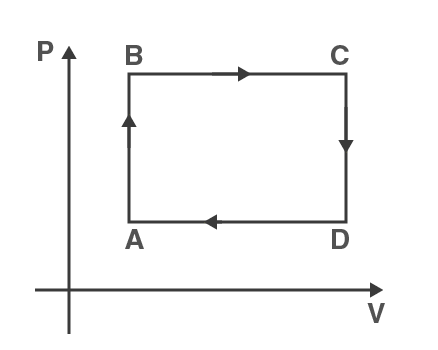The heat given to the system for path ADC is;

(1) 100 J

(2) 80 J

(3) 40 J

(4) 60 J

Solution:

Change in Int energy should be the same;

ΔU = 60 – 30 = 30

In process ABC

ΔQ = ΔU + Δw

ΔQ = 30 + 10

ΔQ = 40 J

3. Initially, an object is kept at a distance of 10 cm from the convex lens and a sharp image is formed at 10 cm ahead of the lens on the screen. Now a glass plate of µ = 1.5 cm and thickness 1.5 cm is placed between object and lens.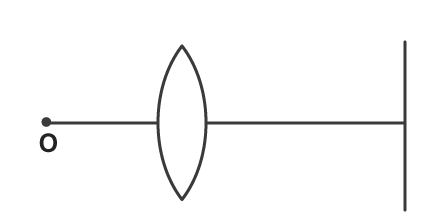The distance by which the screen be shifted to get a sharp image on the screen will be:

(1) $\frac{9}{5}cm$

(2) $\frac{5}{9}cm$

(3) 1 cm

(4) 5 cm

Answer: (2) $\frac{5}{9}cm$

Solution:

ϑ = μ = 2f ⇒ f = 5cm

$s = t(1-\frac{1}{\mu}) = 1.5(1-\frac{1}{312}) = 5$

S is positive ⇒ along the incident ray

U’ = 9.5 cm, ϑ = ?, f = 5 cm

$\frac{1}{\Theta} = \frac{1}{f}+ \frac{1}{u}$

⇒ ϑ’ = $\frac{uf}{U + f} = \frac{(9.5)(5)}{-9.5+5} = \frac{47.5}{4.5}$

Shift = ϑ1 – ϑ = 5 / 9

4. A planet of mass m having angular momentum L is revolving around the sun. The aerial velocity for the planet will be;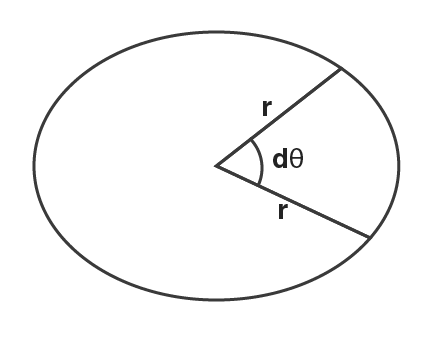(1) $\frac{L}{m}$

(2) $\frac{L}{2m}$

(3) $\frac{2L}{m}$

(4) $\frac{L}{4m}$

Solution:

For small $d\theta\: dA = \frac{1}{2}r^{2}d\theta$ $\frac{dA}{dt} = \frac{1}{2}r^{2}d\theta = \frac{1}{2}r^{2}w = \frac{L}{2m}$

Because, L = mr2w

5. The velocity of a particle $\vec{v}$ at any instant is $\vec{v} = y\hat{i} + x\hat{j}$. The equation of trajectory of the particle is:

(1) x2 + y2 = constant

(2) y2 = x2 + constant

(3) xy = constant

(4) None of these

Solution:

ϑ = $yi + x\hat{j}$ $\frac{dx}{dt} = y \Rightarrow xdx = ydy$ $\frac{dy}{dt} = x \Rightarrow \frac{x^{2}}{2}+\frac{y^{2}}{2}= c$

x2 – y2 = K

6. Initially block of mass M is at rest on a frictionless floor and the spring is in relaxed condition. A constant force is applied on the block. The maximum velocity of block is:

(1) $\frac{F}{\sqrt{mk}}$

(2) $\frac{2F}{\sqrt{mk}}$

(3) $\frac{F}{2\sqrt{mk}}$

(4) $\frac{F}{\sqrt{2mk}}$

Solution:

ϑmax = A w

ϑmax = $\frac{F}{K}\sqrt{\frac{K}{m}}$

ϑmax = $\sqrt{\frac{F}{Km}}$

7. Magnetic field at point O is?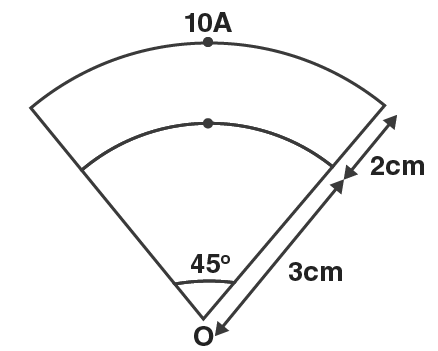(1) 1.5 x 10-5

(2) 10-5

(3) 2 x 10-5

(4) 10-4

Solution:

BO = BAB + BBC + BCD + BOA

BO = $O + \frac{\mu oI\Theta }{4\pi r_{BC}} + O + \frac{\mu oI\Theta }{4\pi r_{OA}}$

Substituting values we get BO = 10-5

8. Charge Q is uniformly distributed over a ring of radius R. The height h, on the axis of the ring at which electric field is maximum?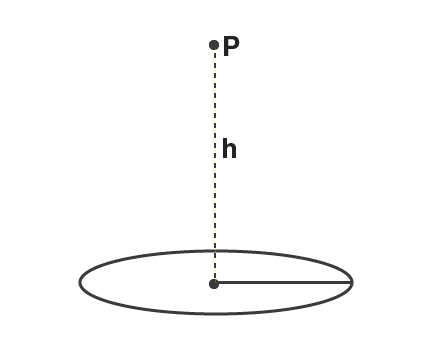(1) $\frac{R}{\sqrt{2}}$

(2) $\frac{R}{2}$

(3) R

(4) None of these

Solution:

E (along) axis of circular coil = $\frac{kQh}{(h^{2}+ R^{2})^{\frac{3}{2}}}$

For the max value of $E\frac{dE}{dH} = O$

Differentiating E w.r.t.h and equating it to O

We get $h = \frac{\pm R}{\sqrt{2}}$

9. Two radioactive elements A & B have initial activity 10 curie & 20 curie respectively. If A has twice the number of moles as that of B. The decay constant λA and λB can be;

(a) (10, 5)

(b) (5, 20)

(c) (20, 10)

(d) (50, 100)

Solution:

Conceptual; λN = A

$\frac{\lambda_{A}}{\lambda_{B}}\frac{N_{A}}{N_{B}} = \frac{A_{A}}{A_{B}}$ $\frac{N_{A}}{N_{B}} = 2 \Rightarrow \frac{\lambda_{A}}{\lambda_{B}}=\frac{\lambda_{A}}{4}$

10. A conducting loop of resistance 10Ω and area is placed in uniform and time-varying magnetic field B = 0.4 sin (50πt). The Charge passing through the loop in t = 0 to t = 10 ms is:

(1) 140 µC

(2) 70 µC

(3) 280 µC

(4) 100 µC

Solution:

$Q = \frac{\Delta\phi}{R}$ $\epsilon = \frac{d\phi}{dt}$ $iR = \frac{d\phi}{dt}$ $\int dQ = \int Rd \phi$ $Q = \frac{1}{R}\int dQ$ $Q = \frac{1}{R}A\int_{0}^{10ms}B\, dt$ $Q = \frac{1}{R}3.5\times10^{-3}\int_{0}^{10ms}0.4si\pi n (50t)\, dt$

Q = 140 μc

11. If current in a current carrying wire is 1.5A, the number of free electrons per unit volume is 8 X 1028m3and area of the cross section is 5 mm2. Drift velocity of electrons will be;

(1) 0.2 mm/s

(2) 2 mm/s

(3) 0.2 mm/s

(4) None of these

Solution:

i = e n A Vd (relation between i and vd)

$V_{d} = \frac{i}{enA} = \frac{1.5}{1.6\times 10^{-9} \times 5\times 10^{-6}\times 8 \times 10^{28}} = 0.02$

12. If temperature difference T1 – T2 is 120°C. The temperature difference between points A and B is?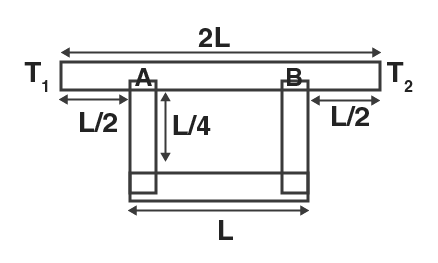(1) 30

(2) 45

(3) 60

(4) 75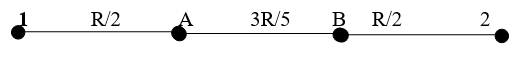Solution:

Effective resistance between A and B is

$\frac{\frac{3R \times 1^2}{2}}{\frac{3R+2R}{2}}=\frac{3R^2}{5R}$

Effective resistance between 1 and 2 is

$\frac{R}{2}+\frac{R}{2}+\frac{3R}{5}$ $R + \frac{3R}{5} = \frac{8R}{5}$ $\frac{T_{A}-T_{B}}{\frac{3R}{5}} = \frac{T_{1}-T_{2}}{\frac{8R}{5}}$

13. Three blocks m, m and M are kept on a frictionless floor as shown in figure. The leftmost block is given velocity v towards right. All the collisions between the blocks are perfectly inelastic. The loss in kinetic energy after all the collisions is 5/6th of initial kinetic energy. The ratio of M/m will be:(1) 1/8

(2) 1/4

(3) 4

(4) 2

Solution:

$\frac{mu}{2m+M} = V_{f}$ $\Rightarrow K_{f} = \frac{1}{6}K_{1}$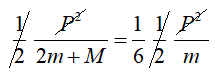6m = 2m + M = 4m = M

14. In mixture 2 mole of He and 1 mole of Ar is present. Find $\frac{(V_{RMS})_{He}}{(V_{RMS})_{Ar}}$ at 300 k.

(1) 6.32

(2) 1.58

(3) 3.16

(4) 10

Solution:

$V_{RMS}\: \alpha \sqrt{m}$ ${V_{RMS}}^{2}\sqrt{\frac{3RT}{M}}$

15. Light of wavelength λ1 = 340 nm and λ2 = 540 nm are incidents on a metallic surface. If the ratio of the speed of the electrons ejected is 2. The work function of the metal is;

(1) 1 eV

(2) 1.85 eV

(3) 1.5 eV

(4) 2 eV

Solution:

$\frac{hc}{\lambda _{1}} = \phi + \frac{1}{2}m(mv)^{2}$ …………..1

$\frac{hc}{\lambda _{2}} = \phi + \frac{1}{2}mV^{2}$ …………..2

Substituting 2 in 1

$\frac{hc}{\lambda _{1}} = \phi + 4 \left [\frac{hc}{\lambda _{2}}-4\right ]$ $3\phi = 4 \frac{12375}{5400}-\frac{12375}{3400}$ $\phi = 1.838 \sim 1.85$

16. Value of i1 (Ampere) when the switch is closed is.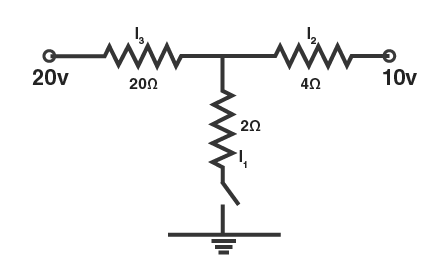(1) 2

(2) 5

(3) 10

(4) 1

Solution:

$\frac{v_{o} – 20}{2} = \frac{v-10}{4} = \frac{v-0}{2}$

⇒ V = 10

$i = \frac{10}{2} = 5A$

17. If the net force on charge kept at O is zero. The value of charge q is :(1) $\frac{+Q}{2}$

(2) $\frac{-Q}{2}$

(3) $\frac{+Q}{4}$

(4) $\frac{-Q}{4}$

Solution:

Net force of q = 0

=> $\frac{KQq}{\frac{d}{2}_2} + \frac{KQq}{d^2}$ = 0

Therefore, q = -Q/4

18. If the value of electric field E = 6.3 x 1027 volt/m for an electromagnetic wave. The value of magnetic field B will be:

(1) 5 x 10-19 T

(2) 2.1 x 1019 T

(3) 5 x 10-20 T

(4) 2.1 x 1020 T

Solution:

Hint: E/B = C

=> B = E/C

19. Two coherent light sources having intensity I1 and I2. If the ratio of is $\frac{I_{max}}{I_{min}}$ 6 : 1. Find $\frac{I_{1}}{I_{2}}$?

(1) $\frac{16}{9}$

(2) $\frac{9}{16}$

(3) $\frac{4}{1}$

(4) $\frac{25}{9}$

Solution:

$\frac{I_{max}}{I_{min}} = \frac{(\sqrt{I_1}+ \sqrt{I_2})^2}{(\sqrt{I_1}- \sqrt{I_2})^2}$

On Simplifying above expression, we get,

$\frac{I_{1}}{I_{2}}$ = $\frac{25}{9}$

20. If length of resistance wire is increased by 0.5 % keeping the volume constant then change in resistance will be;

(1) 0 %

(2) 1%

(3) 0.5%

(4) 2%

Solution:

R = $\frac{\rho l \times l}{a \times l} = \frac{\rho l^2}{V}$

R ∝ l2

$l \omega \times \frac{\Delta R}{I^2} = 2(\frac{\Delta l}{l} \times 100)$

= 2(0.5)

100 x $\frac{\Delta R}{I^2}$ = 1%

21. A uniform L shaped rod each of side A is held as shown in the figure. The angle θ such that the rod remains stable will be.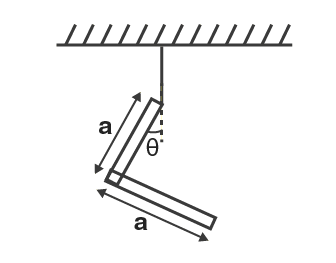(1) $tan^{-1}(\frac{1}{2})$

(2) $tan^{-1}(\frac{1}{3})$

(3) tan-1 (2)

(4) tan-1 (1)

Solution:

Clockwise torque = anti clockwise torque

θ = tan-1 (1/3)

22. A rod of acted by two equal forces as shown in the figure. The coefficient of thermal expansion of the rod is α and area of cross section is A. When the temperature the rod increased by Δ t. The length of the rod does not change. The young’s modulus Y will be.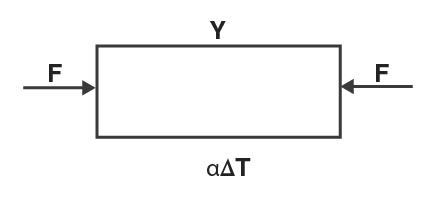(1) $\frac{F}{2A \alpha \Delta T}$

(2) $\frac{F}{A \alpha \Delta T}$

(3) $\frac{2F}{A \alpha \Delta T}$

(4) $\frac{F}{3A \alpha \Delta T}$

$\Delta l = l \alpha$ …(1)

$\Delta l = \frac{Fl}{Ay}$ …(2)

From (1) and (2)

y = $\frac{F}{A \alpha \Delta T}$

23. A capacitor is formed by two square metal-plates of edge a, separated by a distance d. Dielectric of dielectric constants K is filled in the gap as shown in the figure. The equivalent capacitance is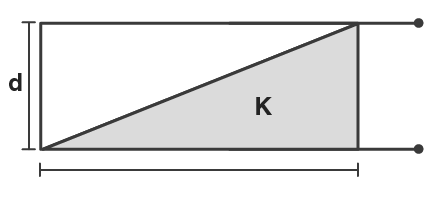(1) $\frac{\kappa \epsilon_0 a^2 lnk}{d(k-1)}$

(2) $\frac{\kappa \epsilon_0 a^2 lnk}{d(k-2)}$

(3) $\frac{\kappa \epsilon_0 a^2 lnk}{2d(k-1)}$

(4) $\frac{2 \kappa \epsilon_0 a^2 lnk}{d(k-1)}$

Solution:

y/d = x/a …(1)

dC2 = $\frac{\epsilon_o a dx}{d-y}$

dC1 = $\frac{K \epsilon_o a dx}{y}$ $dC_{eq} = \frac{dC_1 dC_2}{da+dC_2} = \frac{\epsilon_o a dx}{\frac{C_1}{K} + \frac{d-y}{1} }$ …(2)

$C_{eq} = \int_0^a \frac{\epsilon_o a dx}{\frac{y}{k} + \frac{d-y}{1}}$

After integrating, we get option 1 as an answer.

24. In a semiconductor mobility of electron, i.e. drift velocity per unit applied electric field is 1.6 (S.I unit). Density of electrons is 1019/m3. (Neglect holes concentration). Resistivity of semiconductor is:-

(1) 0.4 Ωm

(2) 2 Ωm

(3) 4 Ωm

(4) 0.2 Ωm

Solution:

$\sigma = ne \mu \Rightarrow \rho = \frac{1}{ne \mu}$ $\frac{1}{10^{19} \times 1.6 \times 10^{-19} \times 1.6}$ = 0.4 Ωm

25. A block of mass M is hanging by a string of negligible mass in a car. The speed of the wave in the string 60 m/s. Now the car is accelerated horizontally by an acceleration at the speed of wave in the string is 60.5 m/s. What is a in terms of g?

(1) $\frac{g}{5}$

(2) $\frac{g}{10}$

(3) $\frac{g}{\sqrt{30}}$

(4) $\frac{g}{30}$

Solution:

v = $\sqrt{\frac{ma}{M}}$

v ∝ $\sqrt{a_{eft}}$ $\frac{\sqrt{g^2+u^2}}{g}$ = 60.5/60

$\frac{g(1+(\frac{a}{g})^2)}{g}$ = 60.5/60

Or (1 + a/2g) = 60.5/60

On simplifying, we have

a = $\frac{g}{\sqrt{30}}$